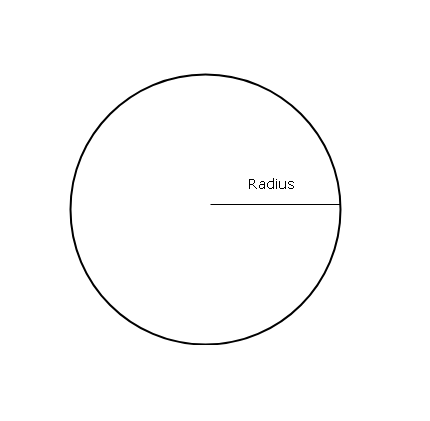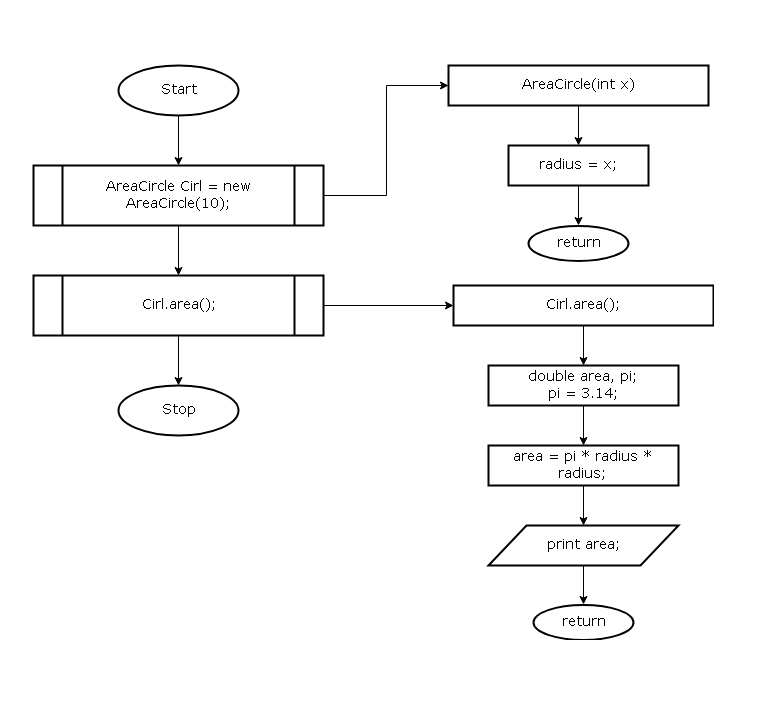## Program to compute area of a circle in Java

The program to compute the area of a circle in Java language is a simple demonstration of evaluating an expression. This formula for an area of a circle is the expression that we need to evaluate to get the expected results.

The program is intended for beginners of Java programming language.

The program is compiled using JDK 8u111 with Netbean IDE 8.2 on a Windows 7 64-bit PC. You can also compile and run the program using Command line utility in Windows.

## Problem Definition

A circle is a basic geometric shape with a radius and a diameter. The diameter length is twice the length of radius.

``diameter = 2 * radius.``

The area of circle is given below.

``Area of a Circle = πr2``## Flowchart – Area of Circle

The public class of this program is AreaCircle() with a constructor that set radius for the circle. Then the method area() computes the area of the circle and prints the output. The method area() is called using an object of class AreaCircle(). The output of the program is given in the next section.Flowchart – Area of Circle

## Program Code

``````class AreaCircle
{

int x;
AreaCircle(int x)
{
}
void area()
{
double area,pi;
pi=3.14;
System.out.println("Area of Circle=" + area);
}

public static void main(String args[])
{

AreaCircle Cir1 = new AreaCircle(10);
Cir1.area();

}
}``````

## Output – Area of Circle

The output of the program for an input ( radius = 10 ) is given below.

``Area of Circle = 314.0``

Related Articles:-